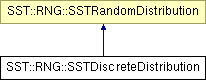# SST::RNG::SSTDiscreteDistribution Class Reference

Creates a discrete distribution for use within SST. More...

`#include "sst/core/rng/discrete.h"`

Inheritance diagram for SST::RNG::SSTDiscreteDistribution:## Public Member Functions

SSTDiscreteDistribution (const double *probs, const uint32_t probsCount)
Creates an exponential distribution with a specific lambda.
SSTDiscreteDistribution (const double *probs, const uint32_t probsCount, SSTRandom *baseDist)
Creates an exponential distribution with a specific lambda and a base random number generator.
~SSTDiscreteDistribution ()
Destroys the exponential distribution.
double getNextDouble ()
Gets the next (random) double value in the distribution.

## Protected Attributes

SSTRandombaseDistrib
Sets the base random number generator for the distribution.
bool deleteDistrib
Controls whether the base distribution should be deleted when this class is destructed.
double * probabilities
The discrete probability list.
uint32_t probCount
Count of discrete probabilities.

## Detailed Description

Creates a discrete distribution for use within SST.

This distribution is the same across platforms and compilers.

## Constructor & Destructor Documentation

 SST::RNG::SSTDiscreteDistribution::SSTDiscreteDistribution ( const double * probs, const uint32_t probsCount ) ` [inline]`

Creates an exponential distribution with a specific lambda.

Parameters:
 lambda The lambda of the exponential distribution

References baseDistrib, deleteDistrib, and probabilities.

 SST::RNG::SSTDiscreteDistribution::SSTDiscreteDistribution ( const double * probs, const uint32_t probsCount, SSTRandom * baseDist ) ` [inline]`

Creates an exponential distribution with a specific lambda and a base random number generator.

Parameters:
 lambda The lambda of the exponential distribution baseDist The base random number generator to take the distribution from.

References baseDistrib, deleteDistrib, and probabilities.

## Member Function Documentation

 double SST::RNG::SSTDiscreteDistribution::getNextDouble ( ) ` [inline, virtual]`

Gets the next (random) double value in the distribution.

Returns:
The next random double from the discrete distribution, this is the double converted of the index where the probability is located

Implements SST::RNG::SSTRandomDistribution.

References baseDistrib, SST::RNG::SSTRandom::nextUniform(), probabilities, and probCount.

The documentation for this class was generated from the following file:

Generated on 14 Sep 2015 for SST by1.6.1